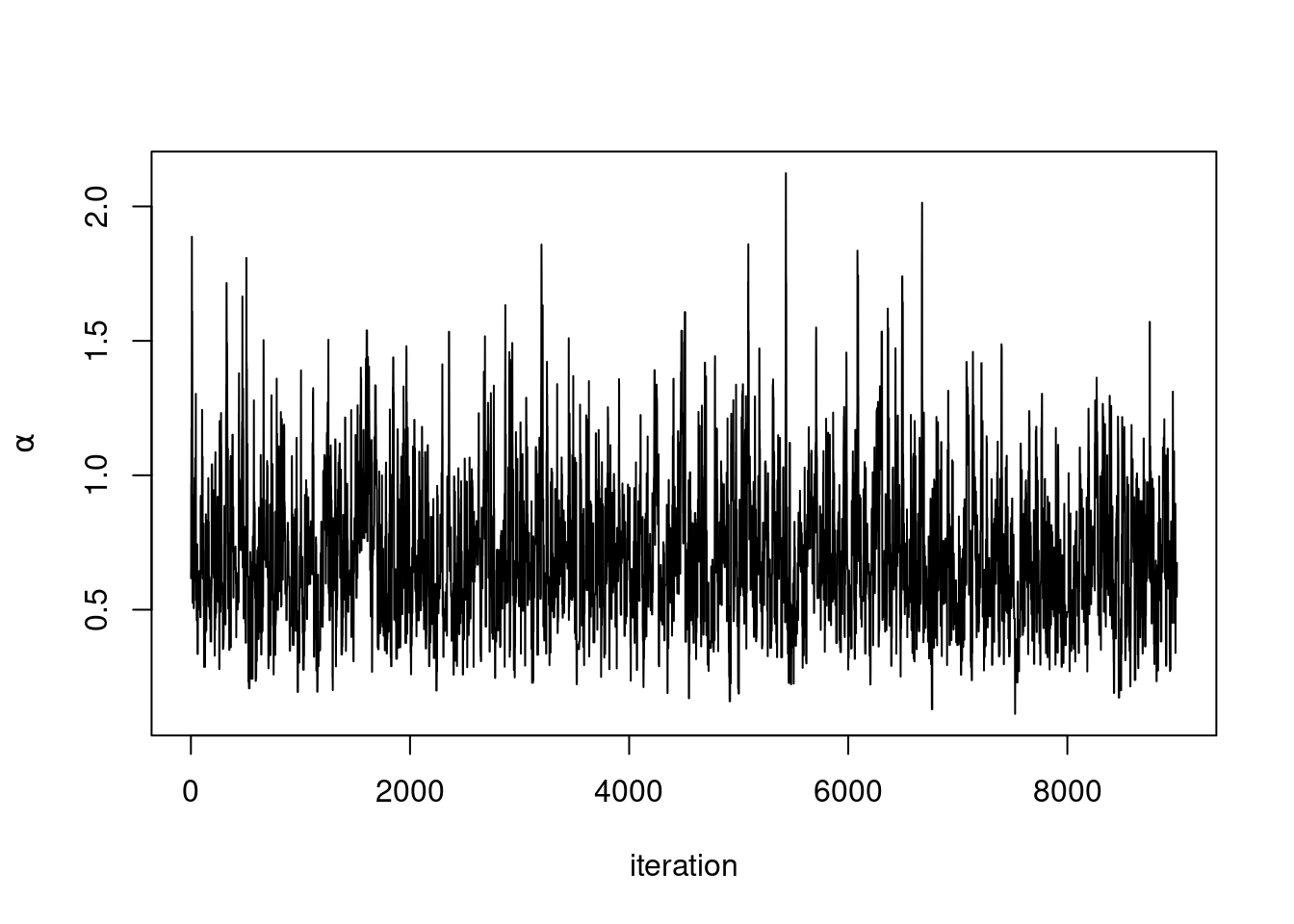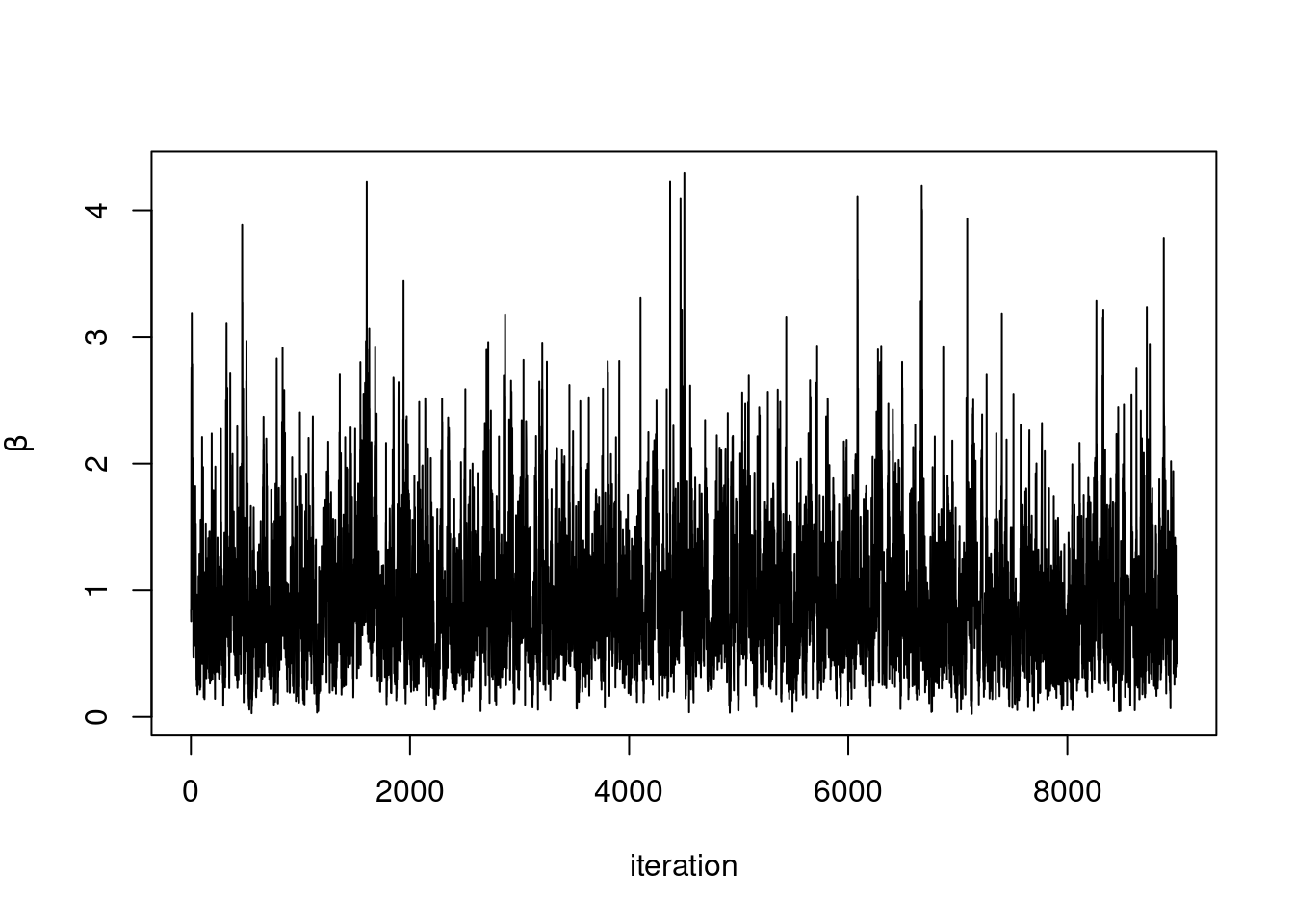# Creating and running a Markov chain Monte Carlo (MCMC) algorithm in NIMBLE

This example shows how to quickly build and run an MCMC algorithm in NIMBLE with default sampler choices.

## Pump example

Let’s use another of the classic WinBUGS examples: the pump model.

A description can be found here. The original example can be found in our GitHub repository here.

## Create the model

We could load the model using `readBUGSmodel()`, but instead we’ll show it fully here:

``library(nimble, warn.conflicts = FALSE)``
``````## nimble version 1.0.1 is loaded.
##
## Note for advanced users who have written their own MCMC samplers:
##   As of version 0.13.0, NIMBLE's protocol for handling posterior
##   predictive nodes has changed in a way that could affect user-defined
##   samplers in some situations. Please see Section 15.5.1 of the User Manual.``````
``````pumpCode <- nimbleCode({
# Define relationships between nodes
for (i in 1:N){
theta[i] ~ dgamma(alpha,beta)
lambda[i] <- theta[i]*t[i]
x[i] ~ dpois(lambda[i])
}
# Set priors
alpha ~ dexp(1.0)
beta ~ dgamma(0.1,1.0)
})

# Create some contrants, data, and initial values to pass to the model builder
pumpConsts <- list(N = 10,
t = c(94.3, 15.7, 62.9, 126, 5.24,
31.4, 1.05, 1.05, 2.1, 10.5))

pumpData <- list(x = c(5, 1, 5, 14, 3, 19, 1, 1, 4, 22))

pumpInits <- list(alpha = 1, beta = 1,
theta = rep(0.1, pumpConsts\$N))``````

## Three ways to run an MCMC

NIMBLE provides three different workflows for running an MCMC. These give you different levels of control over how the algorithm runs. From least to most control, these are: the `nimbleMCMC()` function, the `runMCMC()` function, and the `mcmc\$run()` MCMC object function.

In general, we recommend the `runMCMC()` path, although `nimbleMCMC()` is useful for very straightforward cases.

### `nimbleMCMC()`

The `nimbleMCMC()` function handles everything from model building to compilation to running the MCMC. It takes model code and model inputs (constants, data, and initial values) and returns MCMC samples. The `nimbleMCMC()` function is easy and quick to use but provides little control over the MCMC algorithm. Also, since the objects (the uncompiled and compiled model and MCMC) are used only in the function, they aren’t saved and can’t be manipulated for debugging.

``````nimbleMCMC_samples <- nimbleMCMC(code = pumpCode,
constants = pumpConsts,
data = pumpData,
inits = pumpInits,
nburnin = 1000, niter = 10000)``````
``## Defining model``
``## Building model``
``## Setting data and initial values``
``````## Running calculate on model
##   [Note] Any error reports that follow may simply reflect missing values in model variables.``````
``## Checking model sizes and dimensions``
``## Checking model calculations``
``````## Compiling
##   [Note] This may take a minute.
##   [Note] Use 'showCompilerOutput = TRUE' to see C++ compilation details.``````
``## running chain 1...``
``````## |-------------|-------------|-------------|-------------|
## |-------------------------------------------------------|``````
``head(nimbleMCMC_samples)``
``````##          alpha      beta
## [1,] 0.6171067 0.8436779
## [2,] 0.6703573 0.7550253
## [3,] 0.9662006 1.3331991
## [4,] 0.9662006 2.5562457
## [5,] 1.1742460 2.7611758
## [6,] 1.0969558 1.6755063``````

We can look at the results:

``````plot(nimbleMCMC_samples[ , 'alpha'], type = 'l', xlab = 'iteration',
ylab = expression(alpha))````````````plot(nimbleMCMC_samples[ , 'beta'], type = 'l', xlab = 'iteration',
ylab = expression(beta))``````### `runMCMC()`

The `runMCMC()` function provides more control and a closer look at what NIMBLE is doing. `runMCMC()` takes a NIMBLE MCMC object, so we need to back up and build these things.

First, build the NIMBLE model.

``````pumpModel <- nimbleModel(code = pumpCode, name = 'pump', constants = pumpConsts,
data = pumpData, inits = pumpInits)``````
``## Defining model``
``## Building model``
``## Setting data and initial values``
``````## Running calculate on model
##   [Note] Any error reports that follow may simply reflect missing values in model variables.``````
``## Checking model sizes and dimensions``

Next, build an MCMC object for this model using `buildMCMC()`.

``pumpMCMC <- buildMCMC(pumpModel)``
``````## ===== Monitors =====
## thin = 1: alpha, beta
## ===== Samplers =====
## RW sampler (1)
##   - alpha
## conjugate sampler (11)
##   - beta
##   - theta[]  (10 elements)``````

We can use `runMCMC()` on this uncompiled model if we want to, but we’ll get a warning reminding us that compiled MCMC will be much faster. Uncompiled MCMC will be really slow, but running in R allows easy testing and debugging. Here is how you would run it for 5 iterations:

``runMCMC(pumpMCMC, niter = 5)``
``##   [Warning] Running an uncompiled MCMC algorithm, use compileNimble() for faster execution.``
``## running chain 1...``
``````##      alpha      beta
## [1,]     1 4.0225311
## [2,]     1 2.4588645
## [3,]     1 2.0180446
## [4,]     1 0.9106722
## [5,]     1 1.4839755``````

To compile the model and MCMC object, use `compileNimble()`. Note that the model object must be compiled first for the MCMC to compile.

``CpumpModel <- compileNimble(pumpModel)``
``````## Compiling
##   [Note] This may take a minute.
##   [Note] Use 'showCompilerOutput = TRUE' to see C++ compilation details.``````
``CpumpMCMC <- compileNimble(pumpMCMC, project = pumpModel)``
``````## Compiling
##   [Note] This may take a minute.
##   [Note] Use 'showCompilerOutput = TRUE' to see C++ compilation details.``````

Now we can use `runMCMC()` on the compiled model object.

``runMCMC_samples <- runMCMC(CpumpMCMC, nburnin = 1000, niter = 10000)``
``## running chain 1...``
``````## |-------------|-------------|-------------|-------------|
## |-------------------------------------------------------|``````

We can look at the results:

``plot(runMCMC_samples[ , 'alpha'], type = 'l', xlab = 'iteration',  ylab = expression(alpha))``